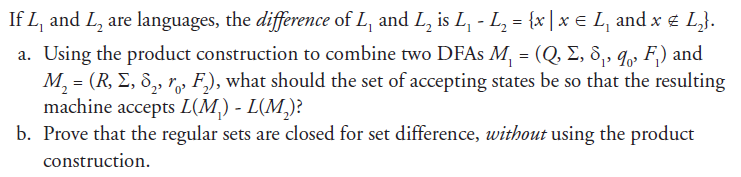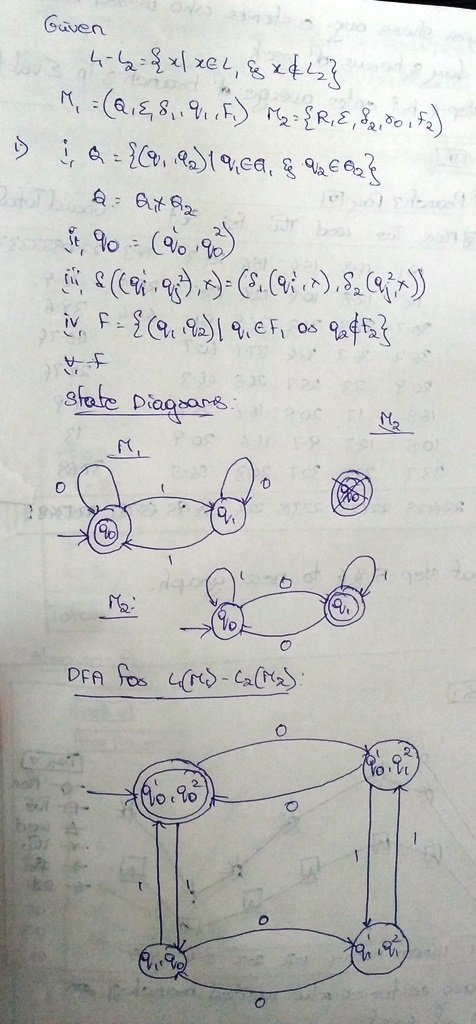# Question & Answer: If L_1 and L_2 are languages, the difference of L_1 and L_2 is L_1 – L_2 = {x|x element l_1 and x notelement L_2}. a. Us…..If L_1 and L_2 are languages, the difference of L_1 and L_2 is L_1 – L_2 = {x|x element l_1 and x notelement L_2}. a. Using the product construction to combine two DFAs M_1 = (Q, sigma, delta_1, q_0, F_1) and M_2 = (R, sigma, delta_2, r_0, F-2), what should the set of accepting states be so that the resulting machine accepts L(M_1)- L(M_2)? b. Prove that the regular sets are closed for set difference, without using the product construction.